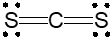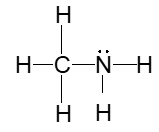Clutch Prep is now a part of Pearson
Ch.10 - Molecular Shapes & Valence Bond TheoryWorksheetSee all chapters

# Electron Geometry

See all sections
Sections
Valence Shell Electron Pair Repulsion Theory
Equatorial and Axial Positions
Electron Geometry
Molecular Geometry
Bond Angles
Hybridization
Molecular Orbital Theory
MO Theory: Homonuclear Diatomic Molecules
MO Theory: Heteronuclear Diatomic Molecules
MO Theory: Bond Order
Molecular Geometry (IGNORE)
VSEPR Theory

The simplest system for geometrical shapes that focuses on the number of electron groups around the central atom.

###### Electron Geometry

Concept #1: Understanding Electron Geometry

The electron geometry of a compound treats surrounding elements and lone pairs on the central element as the same.

Example #1: Determine the electron geometry for the hydrogen sulfide molecule, H2S.

Practice: Determine the electron geometry for the carbon disulfide molecule, CS2A. Linear

B. Bent

C. Trigonal planar

D. Tetrahedral

Example #2: Determine the electron geometry for the following molecule: CH2O

Practice: Determine the number of electron groups for the following cation: AsBr2+

A. 2

B. 3

C. 4

D. 1

Practice: Draw and determine the electron geometry for the following molecule: TeBr4

Practice: Determine the electron geometry of the nitrogen atom within methylamine, CH3NH2.A. Linear

B. Trigonal Pyramidal

C. Tetrahedral

D. Trigonal Planar